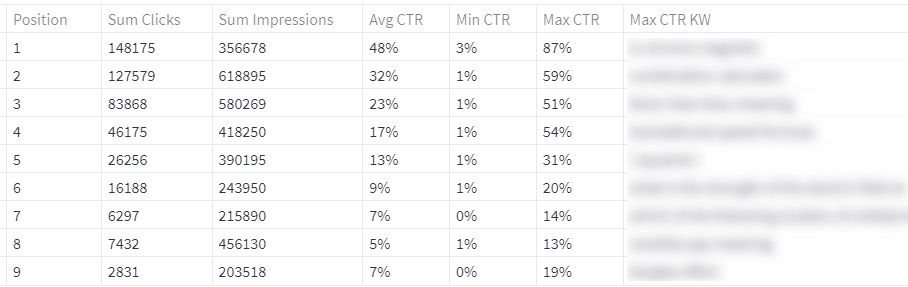Common Topics: data, ctr, int, position, enlighter

Last week SEO Clarity came out with a new SERP CTR study. The numbers were lower than I expected even as an average for all queries. It got me thinking. What is MY average CTR by position? Turns out, it’s much higher. This is likely due to good SEO by optimizing the title, meta, and rich snippets. These calculations can be easily done in Google Sheets, but I wanted to try it in Python and make it an app. Thus this will be a very short tutorial.

In this Python SEO tutorial, we’ll take a standard 1000 record Google Search Console 12-month performance export (Clicks, Impressions, CTR, Position) and sum/mean those metrics by position. Note, if you are a very large site, the 1000 records will only be a snapshot, you’ll want to get much more data via the GSC API before running this. Let’s do it! As always, be careful copying the code as indents are not always preserved.

Not interested in the tutorial? Head straight for the app here!

## Requirements and Assumptions

• Python 3 is installed locally or Google Colab, and basic Python syntax is understood.
• Google Search Console performance data “Queries.csv” export file.

## Import and Install Modules

• pandas: for storing the information in a table form
• NumPy: for easy dataframe type converting

First, we very simply import the 2 modules listed above that the script requires.

```import pandas as pd
import numpy as np```

Next, we read the Google Search Console performance CSV into a pandas dataframe. Note, when you export the data from GSC, it will download a zip file. Unzip and you’ll want to use Queries.csv from that zip.

`df = pd.read_csv("Queries.csv")`

Now we create a counter variable x for a loop coming up, a variable y for you to adjust how many positions you want to process, and create our empty dataframe to store the calculations per SERP position.

```x = 1
y = 9
d = {'Position': [], 'Sum Clicks': [], 'Sum Impressions':[], 'Avg CTR':[],'Min CTR':[],'Max CTR':[],'Max CTR KW':[]}
df2 = pd.DataFrame(data=d)```

Next, we loop by the number of positions you set y to starting with the value of x which is 1 since there technically is no position 0. For each position range x to x.9, we filter the initial dataframe. Sort the dataframe by CTR descending, remove the percent sign so we can process and retype the CTR column from string to float.

```while x < y:
df1 = df[(df['Position'] >=x) & (df['Position'] < x+1)]
df1 = df1.sort_values('CTR',ascending=False)
df1['CTR'] = df1['CTR'].str.replace('%','')
df1['CTR'] = df1['CTR'].astype(np.float16)```

There may be times when you don’t have a keyword in a numerical position, say 11, so we need to use try/except to detect that and keep moving on.  We then simply use the pandas functions, mean(), min(), max(), and sum() to make those calculations along with grabbing the first record’s keyword which will be the highest CTR.

```try:
ctr = int(round((df1['Clicks'].sum()/df1['Impressions'].sum())*100))
ctr_min = int(df1['CTR'].min())
ctr_max = int(df1['CTR'].max())
ctr_max_kw = df1.iloc['Top queries']
clicks = int(df1['Clicks'].sum())
impressions = int(df1['Impressions'].sum())```

Those calculations will result in a default of several decimal places. To remove those, we’ll set the columns to int.

```df2['Avg CTR'] = df2['Avg CTR'].astype(int)
df2['Min CTR'] = df2['Min CTR'].astype(int)
df2['Max CTR'] = df2['Max CTR'].astype(int)
df2['Position'] = df2['Position'].astype(int)
df2['Sum Clicks'] = df2['Sum Clicks'].astype(int)
df2['Sum Impressions'] = df2['Sum Impressions'].astype(int)```

Now we handle positions we don’t have in the data and just pass on through, increasing the counter to get the next position ready and move back up to the start of the loop.

```except:
pass
x += 1```

Once all the positions are processed via the while loop above, we create a dictionary list with all the data for a single position

```   data = {'Position': int((x)),'Sum Clicks':clicks,'Sum Impressions':impressions,'Avg CTR':ctr,'Min CTR':ctr_min,'Max CTR':ctr_max,'Max CTR KW':ctr_max_kw}
df2 = df2.append(data, ignore_index=True)```

For the presentation, we want to add the percent sign, but you can’t if it’s an int value so we convert it to str, and add the percent sign via a simple lambda function. Finally, we display the dataframe.

```df2 = df2.astype(str)
df2['Avg CTR'] = df2['Avg CTR'].apply(lambda x: x + "%")
df2['Min CTR'] = df2['Min CTR'].apply(lambda x: x + "%")
df2['Max CTR'] = df2['Max CTR'].apply(lambda x: x + "%")
df2```

## Sample Output## Conclusion

Now you have the framework to look at your Google Search Console data by position. Lots of future potential to further feature this script out with more calculations and perhaps some data blending from different data sources. Now go supercharge your SEO! Enjoy!

Don’t forget to try the streamlit app here!

Now get out there and try it out! Follow me on Twitter and let me know your Python SEO applications and ideas!